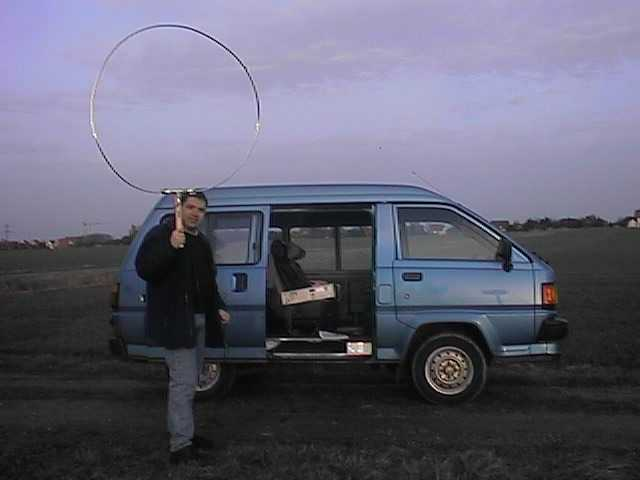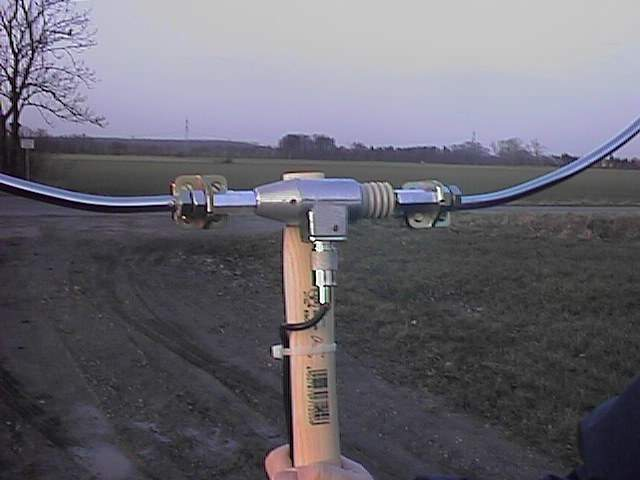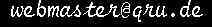DK8KW Longwave Information
Calibration of my Receiving Antenna in dBµV/m

Basic Calibration to convert dBu or dBm readings taken with my receiving equipment into dBµV/m:

Following some instructions given by Dick, PA0SE, I have made a 1 m Diameter loop (for deteils see below) from a solid copper pipe and took some very basic measurements. The comparison of taking the measurments in my shack or out in the fields is marginal.Hen, DF3OE with the loop

I measured the voltage of DCF39 to be -86.9 dBu which is equivalent to a voltage of 35 µV
(0 dBu=0.77V, so -86.9 dBu=20*log(35µV/0.775V)).

From the equation (valid for 137 kHz):

e = 0.00225*D^2*E [Volt]
or

E = e /(0.00225*D^2) [V/m}

E = 35E-6/0.00225 [V/m]
I derive

E = 15 mV/m
equivalent to

E = 83.7  dBµV/m
(83.7 dBµV=20*log(15 mV/1 µV)).

At the same time the signal strength of DCF39 with my regular 18m high umbrella antenna was

-24.4 dBu

This value equals to

- 14.4 dBm
So the correction factor for my antenna system for dBm can be calculated as follows:

- 14.4 dBm + factor = 83.7 dBµV/m

factor = 98.1 dB
and for dBu as follows:

-24.4 dBu+ factor = 83.7  dBµV/m

factor = 108.1 dB.

Conclusions:

For my antenna system and the signal strength given in dBu, add 108.1 dB to achieve values in dBµV/m.Details of my solid loop